# Qlik Design Blog

All about product and Qlik solutions: scripting, data modeling, visual design, extensions, best practices, etc.

Announcements
QlikWorld 2022, LIVE in Denver CO., May 16-19, 2022. REGISTER NOW TO RECEIVE EARLY BIRD PRICINGEmployee

## Set Analysis in the Aggr function

The Aggr() functions is one of the most advanced functions in the QIX engine, and it is not always easy to use. It does not get easier when you put set analysis expressions in it.

In one of my previous posts (Pitfalls of the Aggr function) I recommended having the set analysis expression in both the inner and the outer aggregation function when using set analysis in the Aggr() function. This is a good rule of thumb, because in most cases doing so will generate the result that you want.

But this is not always correct.

In more complex calculations you often need to use the condition in one place only – sometimes in the inner aggregation, sometimes in the outer. It depends on how the condition is formulated. Then it is important to understand the difference between the two positions.

The evaluation of the Aggr() function is a two-step process: In the first step, an intermediary virtual table is created using the inner aggregation and the dimension of the Aggr(). In the second step, this virtual table is aggregated using the outer aggregation.

For example, say that you want to find the largest order value per year. Then you would need to first calculate the sales value per order, and in a second step find the largest of these values. Hence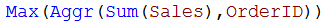The first step aggregates the source data (with multiple records per Order ID) into a virtual table with one record per Order ID, and the second step finds the largest values in the virtual table.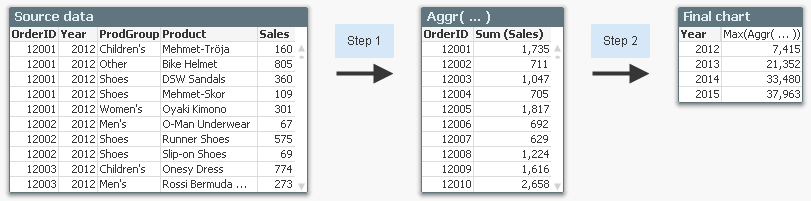However, there is not yet any set analysis in the expression. So, let us use the following requirement instead:

1. Show the largest order value per year
2. Include only products from the product group “Shoes” in the order value
3. Calculate these numbers only for 2014 and 2015

The two conditions correspond to the following set analysis expression: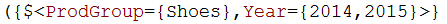But where should this expression be written? In the outer or in the inner aggregation?

To answer this question, we must ask ourselves in which step the conditions should be used. Then it becomes obvious that the condition in product group must be used in step one – in the inner aggregation. If it is used in the outer aggregation only, the order values will be incorrect – they will be calculated from all products.

The condition in year, however, can be put in either place. Hence, the following expression will work fine: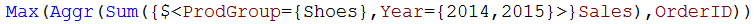From the above example one might draw the conclusion that you always should put the condition in the inner aggregation. But this is not the case. Sometimes you have a condition that cannot be put in the inner aggregation. The following requirement can serve as example:

1. Per year, show the bestselling product within the product group “Shoes”
2. Show how this product ranks compared to all products, also non-shoes

The solution is the following table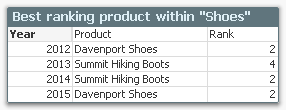The condition in product group should be evaluated in step two, so the expressions used for Product and Rank are:Here it is not possible to have the condition on product group in the inner aggregation, since this would interfere with the calculation of the rank. You must have it in the outer aggregation.

Bottom lines are:

• You need to figure out if your condition should be evaluated in step one (in the inner aggregation) or in step two (in the outer aggregation). This will determine where to put the set analysis expression. You may need to use The Feynman Problem Solving Algorithm.
• If you can’t figure out where to put your set analysis expression – try putting it in both the outer and the inner aggregation function, and keep your fingers crossed. Afterwards you should however verify that the numbers are what you want.

HIC

Further reading related to this topic:

Pitfalls of the Aggr function

A Primer on Set Analysis